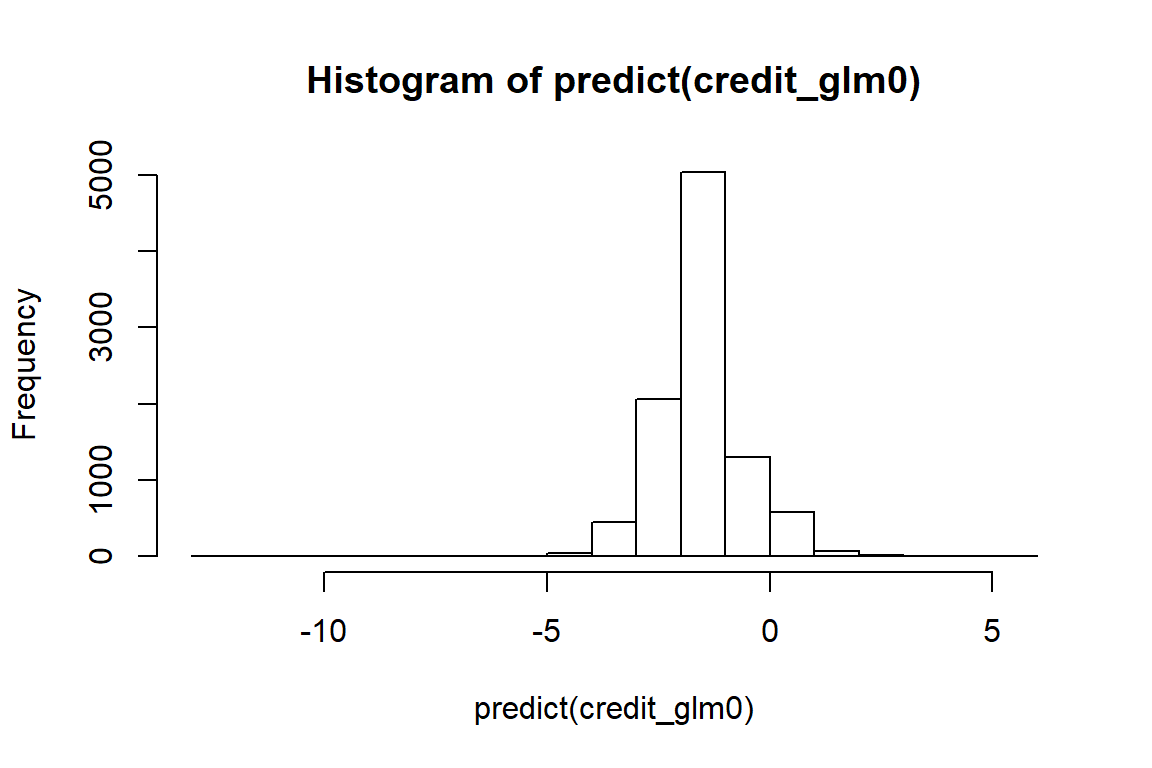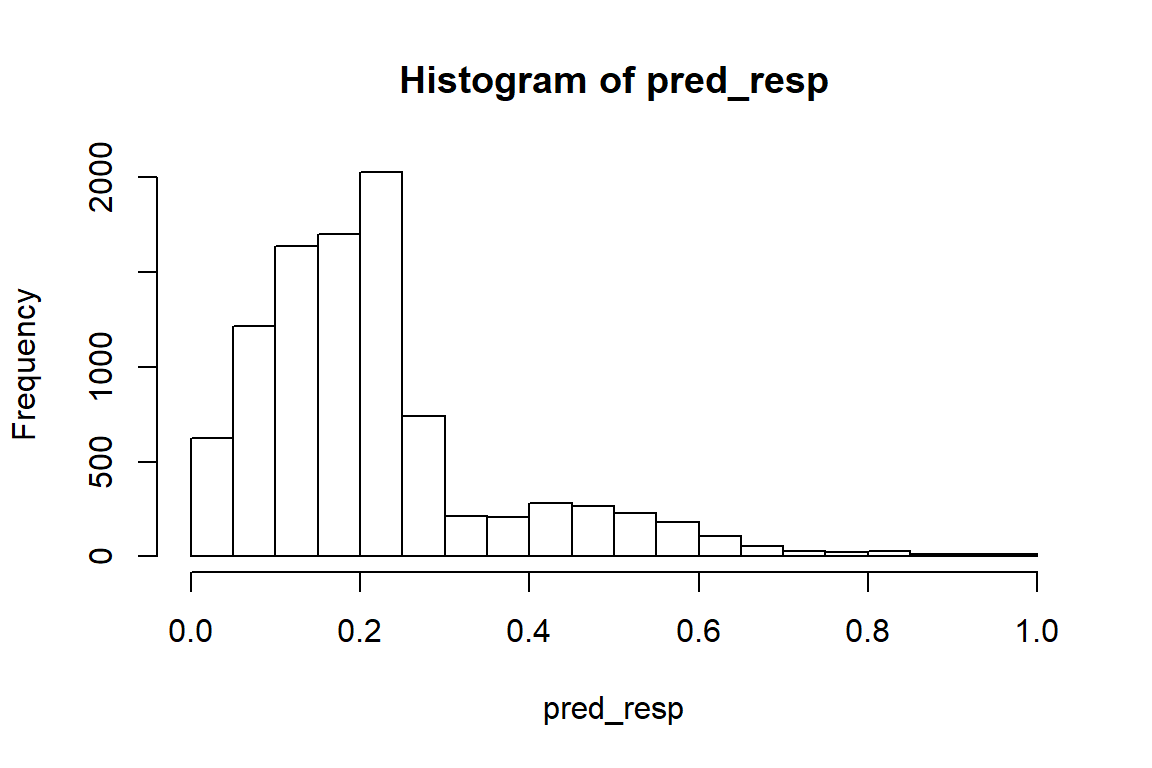# 1 Objective

The objective of this case is to get you understand logistic regression (binary classification) and some important ideas such as cross validation, ROC curve, cut-off probability.

# 2 Credit Card Default Data

We will use a Credit Card Default Data for this lab and illustration. The details of the data can be found at http://archive.ics.uci.edu/ml/datasets/default+of+credit+card+clients. Think about what kind of factors could affect people to fail to pay their credit balance.

We first load the credit scoring data. It is easy to load comma-separated values (CSV).

credit_data <- read.csv(file = "https://xiaoruizhu.github.io/Data-Mining-R/lecture/data/credit_default.csv", header=T)

Look at what information do we have.

colnames(credit_data)
##   "LIMIT_BAL"                  "SEX"
##   "EDUCATION"                  "MARRIAGE"
##   "AGE"                        "PAY_0"
##   "PAY_2"                      "PAY_3"
##   "PAY_4"                      "PAY_5"
##  "PAY_6"                      "BILL_AMT1"
##  "BILL_AMT2"                  "BILL_AMT3"
##  "BILL_AMT4"                  "BILL_AMT5"
##  "BILL_AMT6"                  "PAY_AMT1"
##  "PAY_AMT2"                   "PAY_AMT3"
##  "PAY_AMT4"                   "PAY_AMT5"
##  "PAY_AMT6"                   "default.payment.next.month"

Let’s look at how many people were actually default in this sample.

mean(credit_data$default.payment.next.month) ##  0.2193333 The name of response variable is too long! I want to make it shorter by renaming. Recall the rename() function. library(dplyr) credit_data<- rename(credit_data, default=default.payment.next.month) How about the variable type and summary statistics? str(credit_data) # structure - see variable type summary(credit_data) # summary statistics We see all variables are int, but we know that SEX, EDUCATION, MARRIAGE are categorical, we convert them to factor. credit_data$SEX<- as.factor(credit_data$SEX) credit_data$EDUCATION<- as.factor(credit_data$EDUCATION) credit_data$MARRIAGE<- as.factor(credit_data$MARRIAGE) We omit other EDA, but you shouldn’t whenever you are doing data analysis. go to top # 3 Logistic Regression Randomly split the data to training (80%) and testing (20%) datasets: index <- sample(nrow(credit_data),nrow(credit_data)*0.80) credit_train = credit_data[index,] credit_test = credit_data[-index,] ## 3.1 Train a logistic regression model with all variables credit_glm0 <- glm(default~., family=binomial, data=credit_train) summary(credit_glm0) ## ## Call: ## glm(formula = default ~ ., family = binomial, data = credit_train) ## ## Deviance Residuals: ## Min 1Q Median 3Q Max ## -3.0727 -0.6949 -0.5391 -0.2959 3.2547 ## ## Coefficients: ## Estimate Std. Error z value Pr(>|z|) ## (Intercept) -9.949e-01 1.535e-01 -6.482 9.06e-11 *** ## LIMIT_BAL -8.535e-07 2.833e-07 -3.012 0.002591 ** ## SEX2 -1.246e-01 5.465e-02 -2.280 0.022590 * ## EDUCATION2 -1.317e-01 6.324e-02 -2.082 0.037302 * ## EDUCATION3 -1.886e-01 8.498e-02 -2.220 0.026427 * ## EDUCATION4 -1.104e+00 3.088e-01 -3.576 0.000349 *** ## MARRIAGE2 -1.284e-01 6.171e-02 -2.081 0.037464 * ## MARRIAGE3 -9.189e-02 2.352e-01 -0.391 0.696004 ## AGE 5.010e-03 3.323e-03 1.508 0.131633 ## PAY_0 5.800e-01 3.185e-02 18.210 < 2e-16 *** ## PAY_2 7.624e-02 3.628e-02 2.102 0.035588 * ## PAY_3 3.227e-02 4.049e-02 0.797 0.425396 ## PAY_4 3.898e-02 4.441e-02 0.878 0.380155 ## PAY_5 6.983e-02 4.686e-02 1.490 0.136209 ## PAY_6 2.152e-02 3.968e-02 0.542 0.587592 ## BILL_AMT1 -6.317e-06 2.019e-06 -3.128 0.001759 ** ## BILL_AMT2 5.727e-06 2.601e-06 2.202 0.027684 * ## BILL_AMT3 1.099e-06 2.205e-06 0.498 0.618263 ## BILL_AMT4 -5.726e-06 2.469e-06 -2.319 0.020374 * ## BILL_AMT5 2.661e-06 2.893e-06 0.920 0.357663 ## BILL_AMT6 1.441e-06 2.287e-06 0.630 0.528584 ## PAY_AMT1 -1.020e-05 3.546e-06 -2.878 0.004008 ** ## PAY_AMT2 -5.998e-06 3.251e-06 -1.845 0.065062 . ## PAY_AMT3 7.333e-07 2.783e-06 0.264 0.792159 ## PAY_AMT4 -5.229e-06 2.954e-06 -1.770 0.076678 . ## PAY_AMT5 -2.001e-06 3.016e-06 -0.663 0.507090 ## PAY_AMT6 -2.431e-06 2.100e-06 -1.158 0.246983 ## --- ## Signif. codes: 0 '***' 0.001 '**' 0.01 '*' 0.05 '.' 0.1 ' ' 1 ## ## (Dispersion parameter for binomial family taken to be 1) ## ## Null deviance: 10040.1 on 9599 degrees of freedom ## Residual deviance: 8820.6 on 9573 degrees of freedom ## AIC: 8874.6 ## ## Number of Fisher Scoring iterations: 5 You have seen glm() before. In this lab, this is the main function used to build logistic regression model because it is a member of generalized linear model. In glm(), the only thing new is family. It specifies the distribution of your response variable. You may also specify the link function after the name of distribution, for example, family=binomial(logit) (default link is logit). You can also specify family=binomial(link = "probit") to run probit regression. You may also use glm() to build many other generalized linear models. ### 3.1.1 (Optional) Two-way contingency table and Chi-square test Two-way contingency table is a very useful tool for exploring the relationship between categorical variables. It is essentially the simplest pivot-table (see example below). Often time, after you create a two-way contingency table, Chi-square test is used to test if X affect Y. The null hypothesis is: X and Y are independent (e.g., MARRIAGE has nothing to do with likelihood of default). The $$\chi^2$$ test statistic is defined as $\chi^2=\sum \frac{(observed-expected)^2}{expected},$ where the expected count is calculated by assuming row variable has nothing to do with column variable. Here is a very good tutorial for Chi-square test https://www.youtube.com/watch?v=WXPBoFDqNVk. table_edu <- table(credit_data$EDUCATION, credit_data$default) table_edu ## ## 0 1 ## 1 3399 829 ## 2 4298 1298 ## 3 1501 491 ## 4 170 14 chisq.test(table_edu) ## ## Pearson's Chi-squared test ## ## data: table_edu ## X-squared = 49.19, df = 3, p-value = 1.189e-10 What we saw from above test result is that p-value < 0.05. What is your conclusion? go to top ## 3.2 Get some criteria of model fitting You can simply extract some criteria of the model fitting, for example, Residual deviance (equivalent to SSE in linear regression model), mean residual deviance, AIC and BIC. Unlike linear regression models, there is no $$R^2$$ in logistic regression. # in-sample residual deviance credit_glm0$deviance
##  8820.596
# in-sample mean residual deviance using df
credit_glm0$dev/credit_glm0$df.residual 
##  0.9214035
AIC(credit_glm0)
##  8874.596
BIC(credit_glm0)
##  9068.173

## 3.3 Prediction

Similar to linear regression, we use predict() function for prediction.

To get prediction from a logistic regression model, there are several steps you need to understand. Refer to textbook/slides for detailed math.

1.The fitted model $$\hat{\eta} = b_0 +b_1 x_1 + b_2 x_2 + ...$$ gives you the estimated value before the inverse of link (logit in case of logistic regression). In logistic regression the $$\hat{\eta}$$ are called log odds ratio, which is $$\log(P(y=1)/(1-P(y=1)))$$. In R you use the predict() function to get a vector of all in-sample $$\hat{\eta}$$ (for each training obs).

hist(predict(credit_glm0))2.For each $$\hat{\eta}$$, in order to get the P(y=1), we can apply the inverse of the link function (logit here) to $$\hat{\eta}$$. The equation is $$P(y=1) = 1/ (1+exp(-\hat{\eta}))$$. In R you use the fitted() function or *predict(,type=“response”) to get the predicted probability for each training ob.

pred_resp <- predict(credit_glm0,type="response")
hist(pred_resp)3.Last but not least, you want a binary classification decision rule. The default rule is if the fitted $$P(y=1) > 0.5$$ then $$y = 1$$. The value 0.5 is called cut-off probability. You can choose the cut-off probability based on mis-classification rate, cost function, etc. In this case, the cost function can indicate the trade off between the risk of giving loan to someone who cannot pay (predict 0, truth 1), and risk of rejecting someone who qualifys (predict 1, truth 0).

These tables illustrate the impact of choosing different cut-off probability. Choosing a large cut-off probability will result in few cases being predicted as 1, and chossing a small cut-off probability will result in many cases being predicted as 1.

table(credit_train$default, (pred_resp > 0.5)*1, dnn=c("Truth","Predicted")) ## Predicted ## Truth 0 1 ## 0 7327 191 ## 1 1587 495 table(credit_train$default, (pred_resp > 0.2)*1, dnn=c("Truth","Predicted"))
##      Predicted
## Truth    0    1
##     0 4553 2965
##     1  623 1459
table(credit_train\$default, (pred_resp > 0.0001)*1, dnn=c("Truth","Predicted"))
##      Predicted
## Truth    0    1
##     0    1 7517
##     1    0 2082

# 4 Starter code for German credit scoring

Refer to http://archive.ics.uci.edu/ml/datasets/Statlog+(German+Credit+Data)) for variable description. Notice that “It is worse to class a customer as good when they are bad (weight = 5), than it is to class a customer as bad when they are good (weight = 1).” Define your cost function accordingly!

install.packages('caret')
library(caret) #this package contains the german data with its numeric format
data(GermanCredit)

go to top